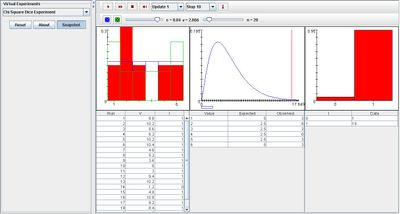# SOCR EduMaterials Activities ChiSquareDiceExperiment

## Description

The Chi Square Fit Experiment is designed to select a random sample from a specified distribution and perform the x^2 goodness of fit test to another specified distribution at a specified level of significance. It corresponds to rolling n dice, each with discrete distributions of {1, 2, 3, 4, 5, 6}. By clicking on the die buttons, the die probability dialog box appears that specifies the sampling and test distributions. With the scroll bars above the graphs, the significance level can be selected from the list box and the sample size n can be varied. The test statistic V and the Chi-Square distribution have approximately 5 degrees of freedom.

In the first graph, the density of the true distribution is shown in blue and the density of the test distribution is shown in green. The sample density graph is also shown in the first graph after each update as red. The density of V and the critical values are shown in blue and the value of the test statistic V is shown in red in the second graph. In the last graph, the empirical density of I is shown in red.

Note that the null hypothesis is rejected if and only if V falls outside of the critical values. Variable I indicates the event that the null hypothesis is rejected.

On each update, the sample values are recoded in the first table. The values of V and I are recorded in the middle table, and the empirical density of I is updated in the last table.

## Goal

To grasp concepts dealing with the chi square distribution those is widely used in theoretical probability distributions and provide an easy and useful simulation to calculate quantities that are approximate to the chi square distribution if the null hypothesis is true.

## Experiments

Go to the SOCR Experiments and select the Chi Square Dice Experiment from the drop-down list of experiments on the top left. The image below shows the initial view of this experiment:

Error creating thumbnail: File missing

When pressing the play button, one trial will be executed and recorded in the distribution table below. The fast forward button symbolizes the nth number of trials to be executed each time. The stop button ceases any activity and is helpful when the experimenter chooses “continuous,” indicating an infinite number of events. The fourth button will reset the entire experiment, deleting all previous information and data collected. The “update” scroll indicates nth number of trials (1, 10, 100, or 1000) performed when selecting the fast forward button and the “stop” scroll indicates the maximum number of trials in the experiment.

When the significance level obtains a high value, the empirical density in the last graph displays higher probability for a success (1). Similarly, when the significance level has a small value, the empirical density in the last graph displays a higher probability for a failure (0). The image below illustrates the Chi Square Dice experiment when the significance level has a high value:Varying parameter n does not change the shapes of the graphs but it does change the numerical values of the expected frequencies in the second table.

The die probability dialog box may be modified to the experimenter’s discretion. By changing the values within the dialog box, the density of the true distribution and the density of the test distribution in the first graph change shape according to the new quantities in the dialog box. Thus, the sample density will also change depending on the density of the true distribution and the density of the test distribution.

By clicking on the blue die and selecting the last choice of values (the most right button), the image below shows the effects when varying values in the dialog box:

## Applications

The Chi-Square Goodness-of-Fit section of the SOCR EBook provides adidtional information on the mathematics and the applications of this statistical methodology.

The Chi Square Dice Experiment is a general simulation that demonstrates the process of using one form of the Chi Square Test—Chi Square Test Goodness of Fit.

It may also be utilized in specialty areas such as marketing. For example, it is important for a marketing manager to know whether the pattern of observed frequencies fit will with the expected values.

More specifically, say researchers are interested to test the hypothesis of a randomly selected group of individuals where the population of men and women are equal. Let the null hypothesis be that men and women have equal probabilities of being chosen. After using this applet, the test will show whether the null hypothesis is tested accurate or not.